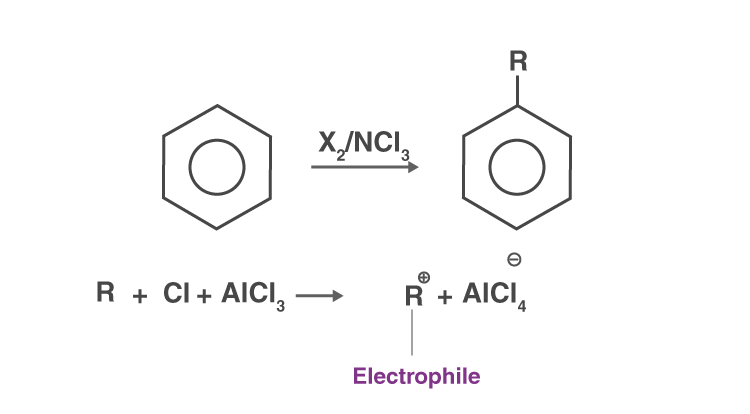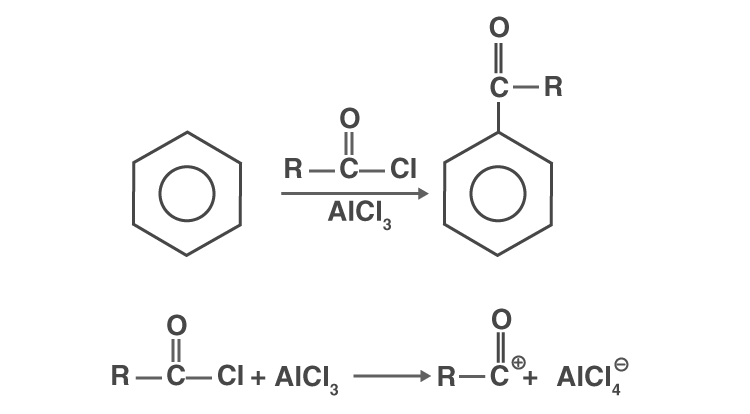# Nucleophilic Substitution Reaction

## What Is Nucleophilic Substitution Reaction?

Nucleophilic substitution reaction is a class of organic reactions where one nucleophile replaces another. It is very similar to the normal displacement reactions which we see in chemistry, where, a more reactive element replaces a less reactive element from its salt solution. The group which takes electron pair and displaced from the carbon is known as “leaving group” and the molecule on which substitution takes place known as “substrate”. The leaving group leaves as a neutral molecule or anion.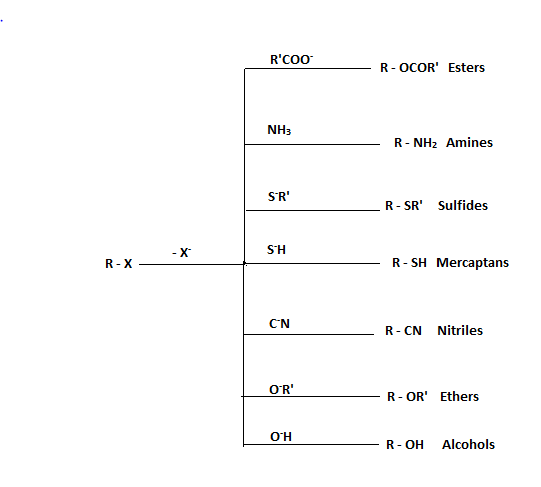In nucleophilic substitution reactions, the reactivity or strength of nucleophile is called as its nucleophilicity. So, in a nucleophilic substitution reaction, a stronger nucleophile replaces a weaker nucleophile from its compound. It can be illustrated roughly as follows.

$R-LG+N{{u}^{\Theta }}\to R-Nu+L{{G}^{\Theta }}$

Where,

R 🡪 Alkyl group

LG 🡪 Leaving group (less nucleophilic)

$N{{u}^{\Theta }}\to$ Stronger nucleophile

Example:

Consider a reaction of methyl bromide with sodium hydroxide, gives sodium bromide as a side product with methanol as the main product.

CH3 – Br + OH → CH3 – OH + Br

Methyl bromide (Substrate)  + Hydroxide ion (Nucleophile)  → Methanol (Product) + Bromide ion

## Nucleophilicity

It is defined as the ability of the nucleophiles to denoted their lone pairs to a positive centre. It is a kinetic term which relates to the rate at which the nucleophile attacks the substrates (R – LG). The nucleophilicity of different nucleophiles can be compared by using the following factors.

### The Basic Strength of Nucleophiles

Basic strength again talks about the ability of a species to donate electron pairs. So the terms basic strength and nucleophilicity are similar and are directly related. The only difference between nucleophilicity and basic strength is that nucleophilicity is a kinetic term whereas, basic strength is a thermodynamic term, meaning, it deals with how much the equilibrium is shifted towards the right for the reaction,

${{B}^{\Theta }}+{{H}^{+}}\to BH$

Where ${{B}^{\Theta }}\to$Base which accepts proton or loses electron.

Therefore generally strong bases are stronger nucleophiles, for example, let us take ${{I}^{\Theta }},B{{r}^{\Theta }},C{{l}^{\Theta }}$ and ${{P}^{\Theta }}$ and compare their nucleophilicities.

For comparing the basic strengths of the above nucleophiles the strength of their conjugate acids are determined and the opposite of that order will be the order of their basic strength (the conjugate base of strong acids are weak bases and vice-versa) and so the order of nucleophilicities can be found.

HF, HCl, HBr and HI are the conjugate acids of ${{F}^{\Theta }},C{{l}^{\Theta }},B{{r}^{\Theta }}$ and ${{I}^{\Theta }}$ respectively. Out of the four acids, the order of acidic strength is as follows

HI > HBr > Hcl > HF (Bond enthalpy). Therefore the order of basic strength and nucleophilicity is ${{F}^{\Theta }}>C{{l}^{\Theta }}>B{{r}^{\Theta }}>{{I}^{\Theta }}$

### Electronegativity of the Nucleophilic Atom

It is a common logic to understand that lone pair of electrons which are loosely held can be denoted easily. Therefore, nucleophiles having lone pairs on highly electronegative atoms are less nucleophilic or weaker nucleophiles.

For example,

$S{{H}^{\Theta }}$ is a better nucleophile than $O{{H}^{\Theta }}$ note that $O{{H}^{\Theta }}$ is a stronger base than $S{{H}^{\Theta }}$. So Basic nature is not the only criteria for deciding nucleophilicities.

### Electron – Releasing Group Near Nucleophilic Centres

Presence of electron-donating groups in the nucleophiles increases their nucleophilicity. For example, $C{{H}_{3}}CO{{O}^{\Theta }}$ is a better nucleophile than $HCO{{O}^{\Theta }}$ because CH3 is an electron releasing group, which increases the electron density on oxygen.

### Steric Hindrance

Highly hindered (crowded) nucleophiles have less ability to move and therefore are weaker nucleophiles. For example, tertiary alkoxide ions are weaker nucleophiles than secondary and primary alkoxide ions due to steric hindrance.

### Charged Or Uncharged

Two nucleophiles having same nucleophilic atom, the one which is charged (Negative) is more nucleophilic than the neutral one because – Negative charge have more affinity towards a positive centre. For example $O{{H}^{\Theta }}$ is a better nucleophile than H2O, even though in both cases oxygen is the nucleophilic atom.

### Polar Solvents Effects

In the case of polar solvents likes, alcohol acids, H2O, etc…., the effect of hydration (solvation) has a role in deciding the nucleophilicities of nucleophiles. The ionic mobility of an ion which is heavily hydrated is highly decreased and therefore, decrease the nucleophilicity.

Hydration is a phenomenon of crowding of water molecules surrounding an ion. Hydration and its effect on nucleophilicity can be explained taking ${{F}^{\Theta }},B{{r}^{\Theta }},C{{l}^{\Theta }}\,and\,{{I}^{\Theta }}$ as examples.

We already determined the order of nucleophilicities of the above nucleophiles wrt to the basic strength but, when these nucleophiles operate in a protic solvent like water, the extent of hydration happening to the nucleophile is also important. The following the two factors on which the extent of hydration depends.

• Charge: Charge α extent of hydration
• Size: Size $\alpha \frac{1}{amount\,as\,hydration}$

(Because a smaller ion can be more easily crowded due to their high charge density)

Therefore, order at which the ions get hydrated is as follows

${{F}^{\Theta }}>C{{l}^{\Theta }}>B{{r}^{\Theta }}>{{I}^{\Theta }}$ (size)

Since ${{F}^{\Theta }}$is heavily hydrated (crowded) in polar solvents ${{F}^{\Theta }}$ is a weakest nucleophile out of the four. Therefore, the order of nucleophilicities of latex]{{F}^{\Theta }},c{{l}^{\Theta }},B{{r}^{\Theta }}\,and\,{{I}^{\Theta }} in protic solvents will be ${{I}^{\Theta }}>,B{{r}^{\Theta }}\,>c{{l}^{\Theta }}>\,{{F}^{\Theta }}$

## Leaving Capacity Of The Leaving Group

In a nucleophile substitution reaction, the rate of the reaction depends on the nucleophilicity of the incoming nucleophile and the leaving capacity of the leaving group which is replaced or substituted. More the leaving capacity of the leaving group, faster the reaction is. The only thumb rule for deciding leaving capacities is that “weaker bases are better leaving group”.

For example, ${{F}^{\Theta }} is the order of leaving capacity.

## Mechanisms Of Nucleophilic Substitution

The rate of nucleophilic substitution reactions not only depends on nucleophiles and leaving capacities but also on the mechanism by which the reaction takes place. There are two mechanisms proposed for nucleophilic substation reactions.

### SN2 Mechanism

It is called substitution nucleophilic bimolecular mechanism. It follows 2nd order kinetics and the rate law for a reaction following the SN2 mechanism is as follows. For SN2 reaction of the form

$R-LG+N{{u}^{\Theta }}\to R-Nu+L{{G}^{\Theta }}$.

$r=K[R-LG][N{{u}^{\Theta }}]$ [R-LG], $[N{{u}^{\Theta }}]$ 🡪 concentration of the substrates and nucleophile respectively.

From the rate law, it is understood that the rate of SN2 reaction depends both on the concentration of the substrate and the nucleophile. Therefore both nucleophilicity of the nucleophile and the leaving capacity of the leaving group increases the rate of the reaction. The mechanism for SN2 reaction is explained taking CH3 – X (methyl halide) and some nucleophile $(N{{u}^{\Theta }})$as an example.

The overall reaction between the two is as follows.

$C{{H}_{3}}-x+N{{u}^{\Theta }}\to C{{H}_{3}}-Nu+{{x}^{\Theta }}$

Mechanism: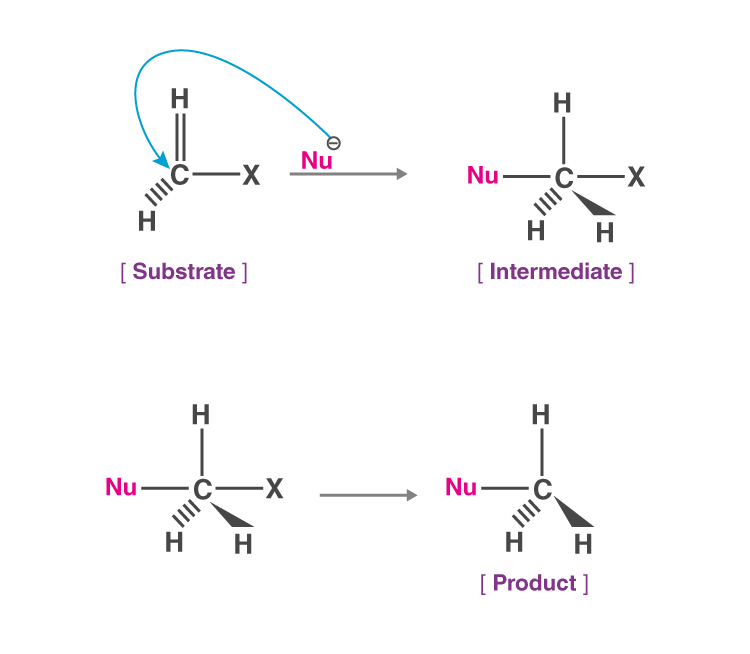It is a single step process consisting of one intermediate. It proceeds through the backside (of the LG) attack of the incoming nucleophile to avoid repulsions, leading to an intermediate which is indicated using two dotted lines between carbon – Nu and carbon-X. The two dotted lines in the intermediate indicate that the C – X bond is broken and the C – Nu Bond is formed simultaneously. And at the end, C – x Bond is completely broken and the c – Nu Bond is completed formed.

Since it’s a backside attack of the nucleophile, the product will always have an inverted configuration wrt the substrate. Therefore, the SN2 mechanism always results in “Inversion of configuration”. In case of t-butyl of chloride, SN2 mechanism becomes increasingly difficult as the nucleophile will find it difficult to attack from the backside due to the presence of bulky methyl.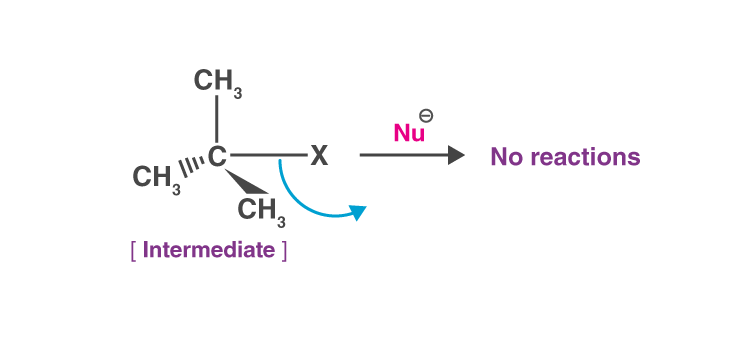Highly crowded substrates will be less reactive towards the SN2 mechanism. The order of reactivity of 1°, 2°, 3° halides is as follows.

1° > 2° > 3° (steric hindrance). The rates of SN2 reactions are enhanced if polar aprotic solvents such as DMF, DMSO, etc are used as reaction mediums.

Example: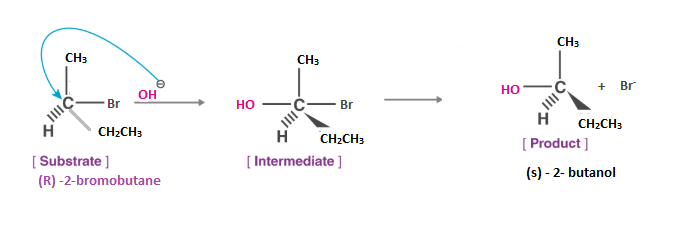### SN2 Transition State

In a transition state of SN2 reaction, a carbon atom and other atoms are in a planar arrangement.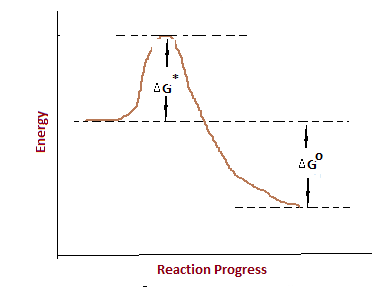### Characteristics of Nucleophilic Substitution Bimolecular Reaction

1. Sensitive to the steric effect.

2. A reaction occurs with inversion of a chiral centre.

3. Methyl halides are more reactive, a primary compound next reactive, secondary halides may react but tertiary halides are not reactive.

4. At C=C in the vinyl compound, there is no reaction.

5. The reaction between the negatively charged nucleophile with the substrate will give a neutral product.

6. Ther reaction between the neutral nucleophile with the substrate will give rise to the positively charged product.

7. A weak base (stable anions) are good leaving groups.

8. Few compounds such as alcohols, fluorides, ether, amines, do not undergo SN2 reactions and also if the leaving group is very small it prevents the SN2 mechanism.

9. The solvents which are capable of donating hydrogen bond will slow down the SN2  reaction because of association with the reactants.

### SN1 Mechanism

It is called unimolecular nucleophilic substitution reaction. For SN1, mechanism having R – X as substrate and $N{{u}^{\Theta }}$ as the incoming nucleophile, the rate law can be presented as follows. R = k [R – x]

Where

r 🡪 rate of SN1 reaction

k🡪 rate constant

{R – X} 🡪 concentration of substrate. From the above equation, we can see that the rate of SN1 mechanism depends only on the concentration of the substrate and is independent of the concentration of the incoming nucleophile. Indirectly, the rate depends on leaving capacity of the leaving group but independent of the nucleophilicity of the incoming nucleophile.

Mechanism: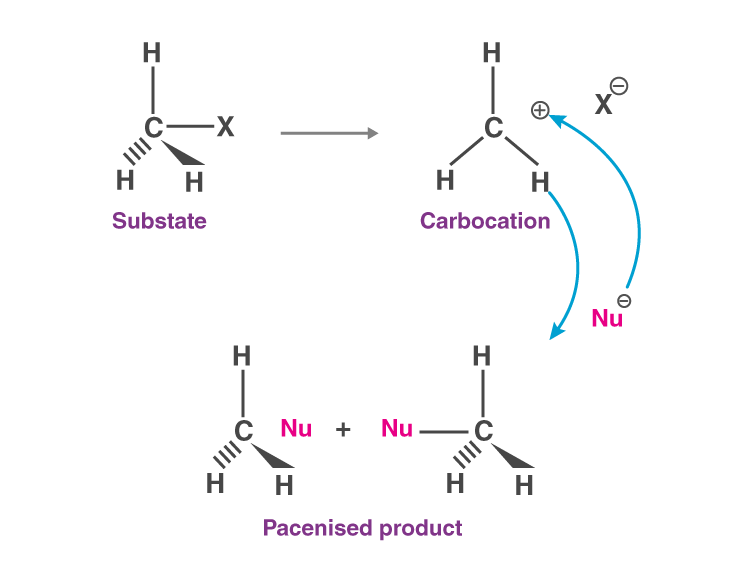Example:

Stereochemistry of SN1 reaction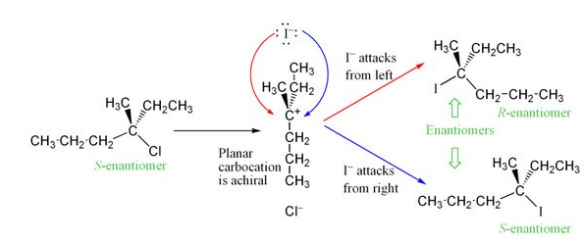It is a two-step process, 1st being the breaking of C – X Bond heterolytically to form a carbocation and ${{X}^{\Theta }}$ and 2nd being the attack of nucleophile onto the carbocation. In a multistep process such as this, the rate-determining step is given by the slowest step and of the two steps above, the formation of the carbocation is the slowest step and therefore the rate-determining step.

Here, unlike SN2, the incoming nucleophile can attack from both sides (opp and the same side as LG). Therefore, the product will always be a racemized product (50% – 50% enantiomers of the same compound).

For different substrates, the rate of SN1 depends linearly on the stability of carbocation formed. Therefore the order of reactivity of 1°, 2°, 3° alkyl halides will be

3° > 2° > 1° (stability of carbocation).

Rate is increased when a polar protic solvent is used since it increases the ionisation to form carbocations

### Characteristics of an SN1 reaction

1. In SN1 mechanism tertiary alkyl halides are more reactive.

2. The mechanism depends on the stability of the carbocation.

3. In SN1 mechanism better-leaving groups are larger halide ions.

OH– < Cl < Br < I

4. In this mechanism, the nature of the nucleophile does not matter much because nucleophilic addition takes place after the formation of a carbocation.

5. Rate of the reaction is controlled by stabilizing the carbocation which stabilizes the transition state of the reaction.

## Comparison of the SN1 and SN2 reaction

 SN1 SN2 Stepwise mechanism forms a carbocation intermediate A one-step mechanism. The rate-determining step involves a single molecule The rate-determining steps involve 2 molecules. It involves rearrangements of carbocations No rearrangements of the carbocation. Compared to the reactant’s product have both inverted and retained configuration. Compared to the reactant’s products will have an inverted configuration. Reactivity order: 30 > 20 > 10> methyl Reactivity order: 30 < 20 < 10< methyl

## Electrophilic Substitution Reactions

As the name suggests, in these type of reactions, one electrophile (positive) charge replaces another electrophile. It’s usually seen in the ring substitutions of benzene and its derivatives. The mechanisms of electrophilic substitution in benzene can be illustrated as follows.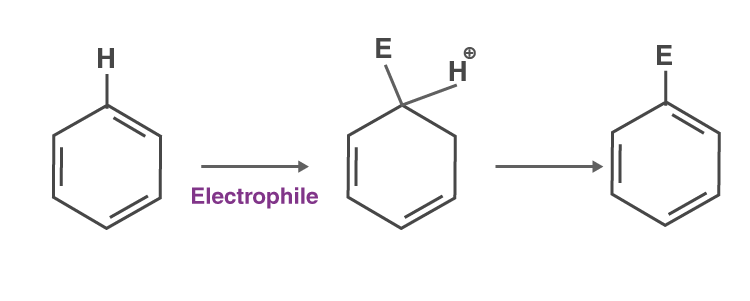Knowledge of different types of electrophiles produced during different electrophilic substitution reactions is important.

## Nitration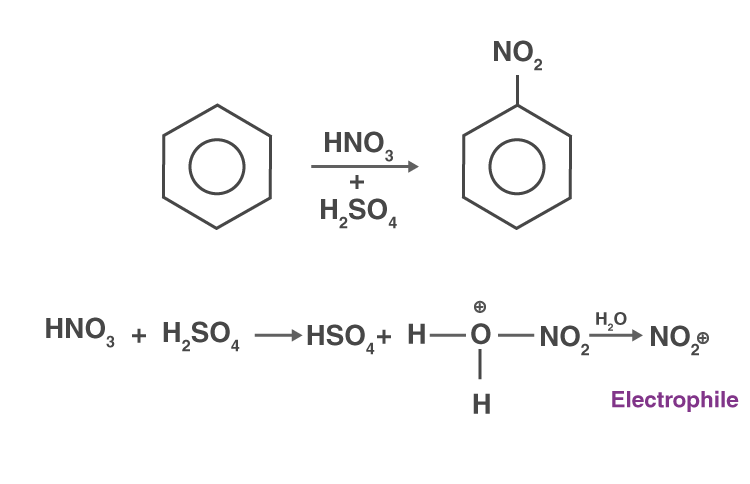## Friedel Crafts Halogenation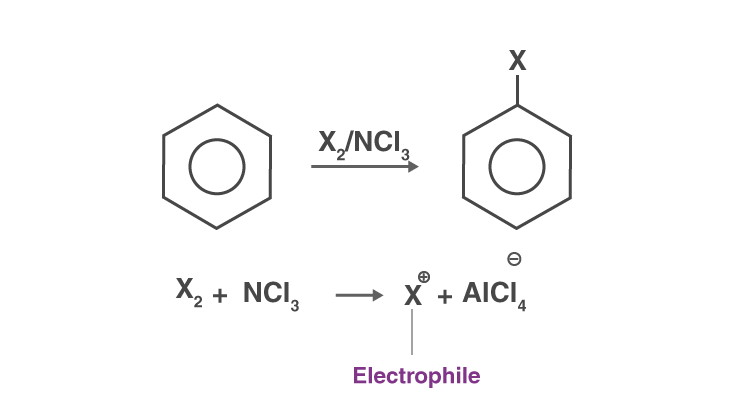## Friedel Crafts Alkylation